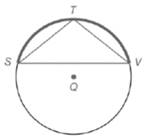Chapter 6.1, Problem 39EElementary Geometry For College St...

7th Edition
Alexander + 2 others
ISBN: 9781337614085

Solutions

Chapter
SectionElementary Geometry For College St...

7th Edition
Alexander + 2 others
ISBN: 9781337614085
Textbook Problem

If S T ⌢ ≅ T V , ⌢ explain why Δ S T V is an isosceles triangle.To determine

To explain:

The statement “If STTV, why ΔSTV is an isosceles triangle”.

Explanation

Given:

The provided statement is “If STTV, ΔSTV is an isosceles triangle”.

Theorem used:

The measure of an inscribed angle of a circle is one-half the measure of its intercepted arc.

Calculation:

We have STTV.

From the figure we see that ST and TV are intercepted arcs of V and S respectively

Still sussing out bartleby?

Check out a sample textbook solution.

See a sample solution

The Solution to Your Study Problems

Bartleby provides explanations to thousands of textbook problems written by our experts, many with advanced degrees!

Get Started

A sample with a mean of M = 8 has X = 56. How many scores are in the sample?

Essentials of Statistics for The Behavioral Sciences (MindTap Course List)

Change 4500 ft/h to ft/min.

Elementary Technical Mathematics

Find the value of the sum. 29. i=1n2i

Single Variable Calculus: Early Transcendentals, Volume I

Evaluate the integral. 25. tan4xsec6xdx

Single Variable Calculus: Early Transcendentals

For y=12gt2 (where g is a constant), dydt=. a) 14g2 b) 12g c) gt d) gt2

Study Guide for Stewart's Single Variable Calculus: Early Transcendentals, 8th